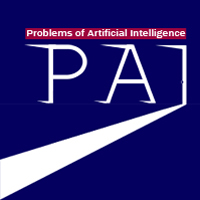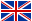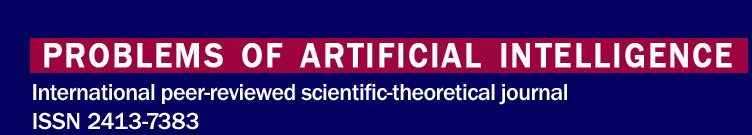﻿ Problems of Artificial Intelligence № 4(11)'2018РУСENG

# Problems of Artificial Intelligence № 4(11)'2018

About

For Authors

Archive

Contacts

Search

UDC 519.7
A. N. Kurganskiy. ON AN ALGORITHMIC MODEL OF RELATIVITY
In the article the task of authentication of parameter is examined as a continuous function of parabolic equalization is in partials. Analytical expression is found for the calculation of gradient of the non–obvious set functional. Gradient is used to determine the modernized classical method of Lagrange multipliers.
Keywords: finite state automata, interacting of automata, relativity.

UDC 681.5.09
V.I. Levin. CONTINUOUS-LOGICAL METHODS OF CALCULATING OF RELIABILITY OF COMPLEX SYSTEMS. II. CALCULATION OF SOME CLASSES OF SYSTEMS
Precise and approximate methods of analyzing the reliability of complex technical systems are described, using the apparatus of logical determinants. Analytical calculated relations are derived for some classes of systems (symmetric, equilibrium threshold, nonequilibrium threshold).
Keywords: complex system, switching process, reliability process, dynamic automaton, binary operator, operator structure, logical reliability theory.

UDC 004.93'1; 004.032.26
A. Yu. Maksimova. USING GENERATIVE ADVERSARIAL NET FOR IDENTIFICATION EXAMINATION TASK
This article is the first theoretical part of the work. The classification model based on the generative-adversarial net (GAN) is created for the identification examination task. The formalization of the identification examination task is performed in the form of a classification problem with a negative class. The overview of GAN is made. The improved techniques for semi-supervised learning GAN is described for classification tasks.
Keywords: classification, semi-supervised learning, generative-adversarial net, neural network, identification examination.

UDC 519.21
O. V. Aleksandrova. GROUP ANALYSIS AND ERGODICITY OF STOCHASTIC PROCESSES
In the paper the application of group analysis methods for stochastic differential Ito equations is considered. It is shown that the methods of group analysis of stochastic differential Ito equations make it possible to construct invariant probability measures of processes that do not have stationary distributions. Or lead to such for that one an invariant measure exists for it.
Keywords: stochastic process, group analysis, symmetry, invariant measure.

UDC 004.942; 519.652
O. S. Voronova. GEOMETRICAL MODEL OF DEPENDENCE THE PHYSICAL PARAMETERS OF THE REFRIGERANT R407C
The paper presents and analytically describes a geometric model the physical state of the refrigerant R407C. This model is the dependence of enthalpy on temperature and volume of a substance. The developed geometric model is presented in the form of a two-parameter response surface, which belongs to three-dimensional space. This model is described using the mathematical apparatus of geometric modeling of processes and phenomena – BN-calculation. The analysis of effectiveness the geometric modeling of dependence the parameters enthalpy is presented: some enthalpy parameters were obtained as a result of graphical measurements using the log P-h thermodynamic diagram, other parameters were calculated on the basis of the developed geometric model. As a result, we can conclude that the geometric model is in good convergence with the log P-h thermodynamic diagram.
Keywords: BN-calculation, geometrical model, surface compartment, support contours, forming surfaces, refrigerant parameters.

UDC 004.75:004.021
А. А. Koibash, T. V. Zavadskaya, S. V. Kryvosheev. RESEARCH OF THE EFFECTIVENESS OF NVIDIA CUDA TECHNOLOGIES IN DISTRIBUTED COMPUTER SIMULATORS OF MOVING OBJECTS
The ways of applying the algorithm A * in computer simulators for prediction the trajectory of a moving object are considered in this paper. To improve the performance of the traectory generation, the use of a sorting tree and hash tables was proposed. The study of the algorithm with the use of these structures. The test results showed a significant increase in the speed of the calculations in distributed computer simulators.
Keywords: optimal trajectory generation, A * distributed simulator, sorting tree.

UDC 004.89
V. N. Pavlysh, S. A. Zori,E. I. Burlaeva. THE PROBLEM OF INFORMATION CLASSIFICATIONWHEN DATA BASE FORMING INCOMPUTERTEACHING SYSTEMS
In the article the task of methods analysis and principles choice for text information classification when data base forming during computer teaching system creation is considered. The effectiveness of combinational principle for task solution noting preferences and defects of existing methods during deal with specific information groups is provided.
Keywords: information, text, method, system, effectiveness. principle.